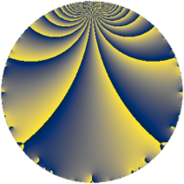# Properties

 Label 475.3.cLevel $475$ Weight $3$ Character orbit 475.c Rep. character $\chi_{475}(151,\cdot)$ Character field $\Q$ Dimension $61$ Newform subspaces $9$ Sturm bound $150$ Trace bound $7$

# Related objects

## Defining parameters

 Level: $$N$$ $$=$$ $$475 = 5^{2} \cdot 19$$ Weight: $$k$$ $$=$$ $$3$$ Character orbit: $$[\chi]$$ $$=$$ 475.c (of order $$2$$ and degree $$1$$) Character conductor: $$\operatorname{cond}(\chi)$$ $$=$$ $$19$$ Character field: $$\Q$$ Newform subspaces: $$9$$ Sturm bound: $$150$$ Trace bound: $$7$$ Distinguishing $$T_p$$: $$2$$, $$7$$

## Dimensions

The following table gives the dimensions of various subspaces of $$M_{3}(475, [\chi])$$.

Total New Old
Modular forms 106 67 39
Cusp forms 94 61 33
Eisenstein series 12 6 6

## Trace form

 $$61q - 114q^{4} - 18q^{6} - 5q^{7} - 157q^{9} + O(q^{10})$$ $$61q - 114q^{4} - 18q^{6} - 5q^{7} - 157q^{9} - 15q^{11} + 174q^{16} - q^{17} + 45q^{19} - 76q^{23} + 138q^{24} - 34q^{26} - 34q^{28} + 68q^{36} + 174q^{38} - 146q^{39} + 30q^{42} - 195q^{43} - 24q^{44} - 39q^{47} + 304q^{49} + 250q^{54} - 190q^{57} + 62q^{58} + 141q^{61} + 116q^{62} + 101q^{63} - 578q^{64} + 244q^{66} + 550q^{68} + 59q^{73} - 276q^{74} - 446q^{76} + 115q^{77} + 905q^{81} - 164q^{82} + 150q^{83} - 574q^{87} + 34q^{92} - 36q^{93} - 742q^{96} + 613q^{99} + O(q^{100})$$

## Decomposition of $$S_{3}^{\mathrm{new}}(475, [\chi])$$ into newform subspaces

Label Dim. $$A$$ Field CM Traces $q$-expansion
$$a_2$$ $$a_3$$ $$a_5$$ $$a_7$$
475.3.c.a $$1$$ $$12.943$$ $$\Q$$ $$\Q(\sqrt{-19})$$ $$0$$ $$0$$ $$0$$ $$5$$ $$q+4q^{4}+5q^{7}+9q^{9}+3q^{11}+2^{4}q^{16}+\cdots$$
475.3.c.b $$2$$ $$12.943$$ $$\Q(\sqrt{-13})$$ None $$0$$ $$0$$ $$0$$ $$10$$ $$q+\beta q^{2}-\beta q^{3}-9q^{4}+13q^{6}+5q^{7}+\cdots$$
475.3.c.c $$2$$ $$12.943$$ $$\Q(\sqrt{19})$$ $$\Q(\sqrt{-19})$$ $$0$$ $$0$$ $$0$$ $$0$$ $$q+4q^{4}-3\beta q^{7}+9q^{9}-3q^{11}+2^{4}q^{16}+\cdots$$
475.3.c.d $$4$$ $$12.943$$ 4.0.7600.1 $$\Q(\sqrt{-95})$$ $$0$$ $$0$$ $$0$$ $$0$$ $$q+\beta _{1}q^{2}+(\beta _{1}-\beta _{2})q^{3}+(-4+3\beta _{3})q^{4}+\cdots$$
475.3.c.e $$4$$ $$12.943$$ 4.0.462080.1 $$\Q(\sqrt{-95})$$ $$0$$ $$0$$ $$0$$ $$0$$ $$q+\beta _{1}q^{2}+(-\beta _{1}-\beta _{2})q^{3}+(-4+\beta _{3})q^{4}+\cdots$$
475.3.c.f $$8$$ $$12.943$$ $$\mathbb{Q}[x]/(x^{8} - \cdots)$$ None $$0$$ $$0$$ $$0$$ $$0$$ $$q+\beta _{1}q^{2}+\beta _{3}q^{3}+(-1+2\beta _{4})q^{4}+\cdots$$
475.3.c.g $$12$$ $$12.943$$ $$\mathbb{Q}[x]/(x^{12} + \cdots)$$ None $$0$$ $$0$$ $$0$$ $$-20$$ $$q+\beta _{1}q^{2}+\beta _{9}q^{3}+(-1+\beta _{2})q^{4}+(-2+\cdots)q^{6}+\cdots$$
475.3.c.h $$14$$ $$12.943$$ $$\mathbb{Q}[x]/(x^{14} + \cdots)$$ None $$0$$ $$0$$ $$0$$ $$-20$$ $$q+\beta _{1}q^{2}-\beta _{4}q^{3}+(-2+\beta _{2})q^{4}-\beta _{5}q^{6}+\cdots$$
475.3.c.i $$14$$ $$12.943$$ $$\mathbb{Q}[x]/(x^{14} + \cdots)$$ None $$0$$ $$0$$ $$0$$ $$20$$ $$q+\beta _{1}q^{2}-\beta _{4}q^{3}+(-2+\beta _{2})q^{4}-\beta _{5}q^{6}+\cdots$$

## Decomposition of $$S_{3}^{\mathrm{old}}(475, [\chi])$$ into lower level spaces

$$S_{3}^{\mathrm{old}}(475, [\chi]) \cong$$ $$S_{3}^{\mathrm{new}}(19, [\chi])$$$$^{\oplus 3}$$$$\oplus$$$$S_{3}^{\mathrm{new}}(95, [\chi])$$$$^{\oplus 2}$$